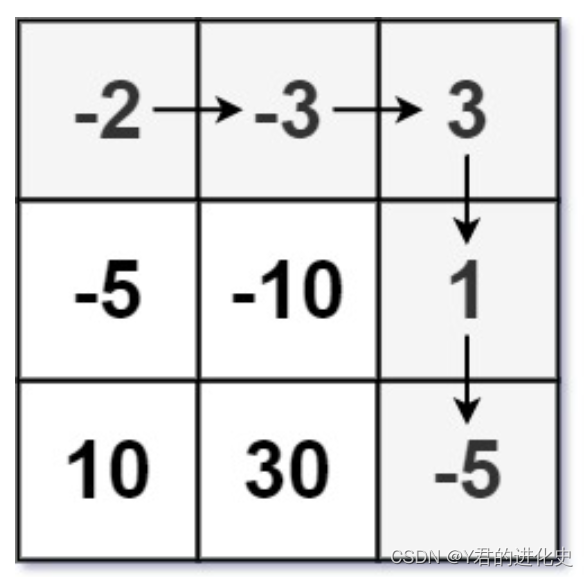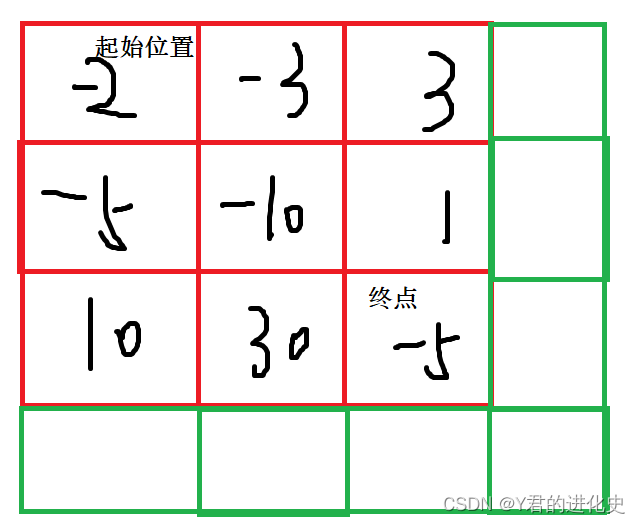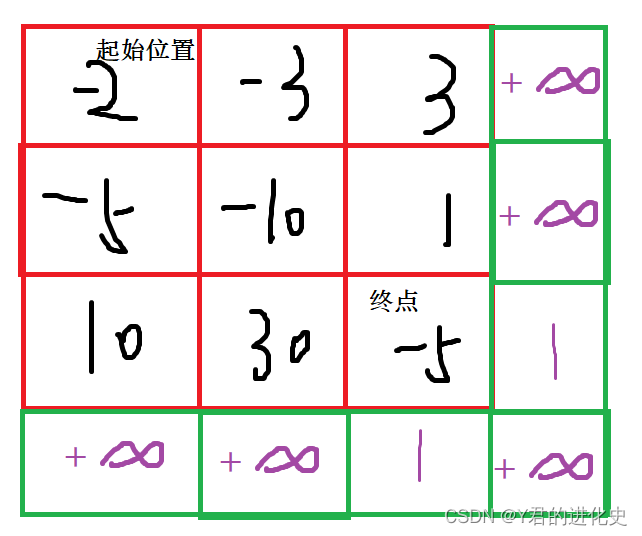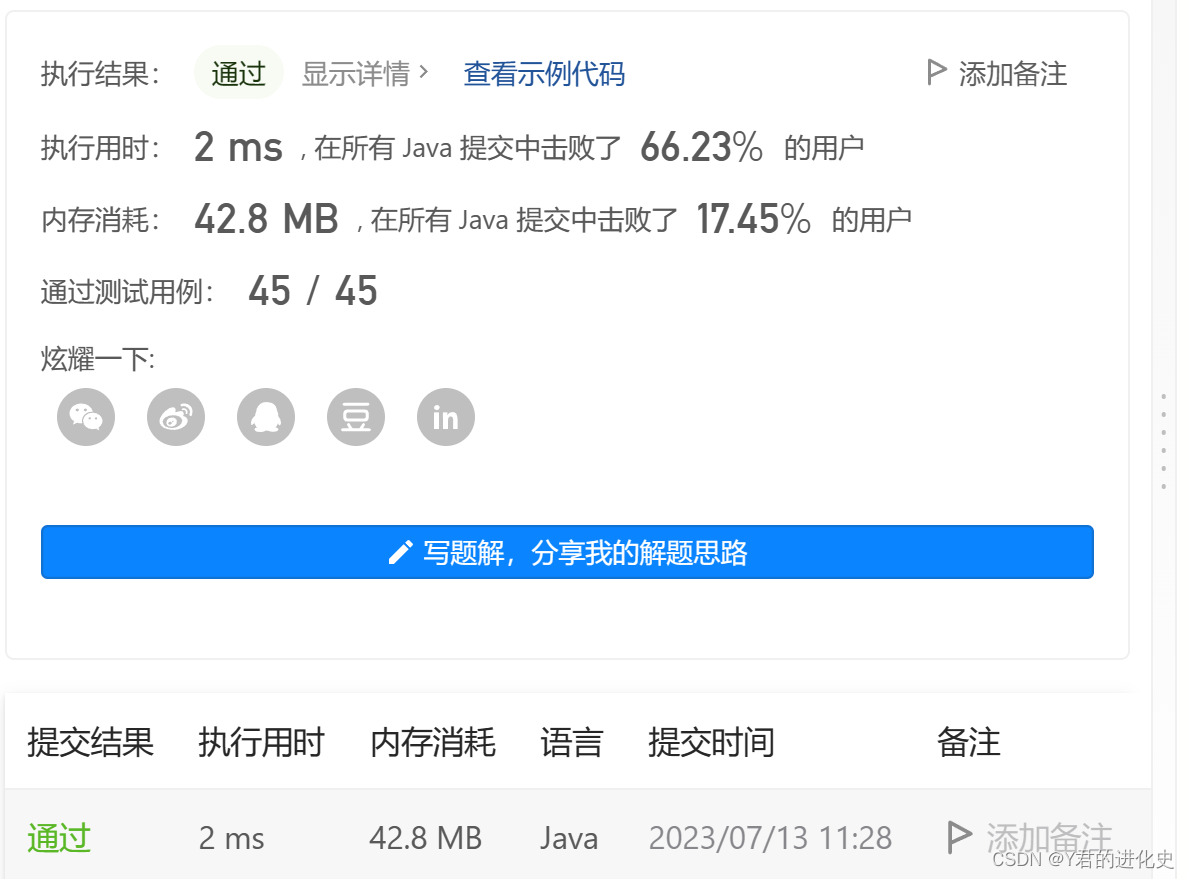##MSIPO技术圈 首页 IT技术 查看内容

# dp算法 力扣174地下城游戏

2023-07-13

174. 地下城游戏 - 力扣（LeetCode）

# 一、题目详情m == dungeon.length
n == dungeon[i].length
1 <= m, n <= 200
-1000 <= dungeon[i][j] <= 1000

# 二、题目解析

1、dp[i][j]表示，从[i][j]位置开始，到终点所需最低初始健康点数；

2、dp[i][j]表示，从位置开始，到[i][j]所需最低初始健康点数.

1. 对于[i][j]位置，它的下一步是[i][j+1] 或者 [i+1][j].
2. 设[i][j]位置所需最低初始健康点数为dp[i][j]， dp[i][j]+dungeon[i][j] >= dp[i][j+1] 以及 dp[i][j]+dungeon[i][j] >= dp[i+1][j]
3. 故 dp[i][j] >= dp[i+1][j] - dungeon[i][j] 或 dp[i][j] >= dp[i][j+1] - dungeon[i][j]填表顺序是从下往上，从右往左，故需考虑虚拟节点存储值大小。我们只需要保证终点结点计算时是使用虚拟结点，其他结点不使用虚拟结点，故将虚拟节点中，影响终点的结点置为1，其余结点置为无穷大。最后返回dp即可。

# 三、代码

``````class Solution {
public int calculateMinimumHP(int[][] dungeon) {
//1.创建dp表
int m = dungeon.length;
int n = dungeon.length;
int[][] dp = new int[m+1][n+1];
//2.初始化
for(int i=0; i<=m; i++){
dp[i][n] = Integer.MAX_VALUE;
}
for(int j=0; j<=n; j++){
dp[m][j] = Integer.MAX_VALUE;
}
dp[m][n-1] = dp[m-1][n] = 1;
//3.填表
for(int i=m-1; i>=0;i--){
for(int j=n-1; j>=0; j--){
dp[i][j] = Math.min(dp[i][j+1],dp[i+1][j])-dungeon[i][j];
dp[i][j] = Math.max(dp[i][j],1);
}
}
//返回值
return dp;
}
}``````